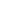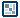## Question of the WeekFlash MX
: Switch / Case
written by ilyas usal a.k.a. pom

Bored with endless if... else ? Flash MX features a new way to deal with it: switch. This instruction already exists in several language (Jscript, PHP, C...) and allows you to evaluate expressions and is used as a substitute to if/else.

#### Here's an example of how it is used : put this in the first frame of a new movie.

Troubleshooting : Be careful that nothing should follow the : in a case
Thus writing case 2:trace("Big big badaboom"); will come out as an error. There must be at least one blank space.

#### How it works:

num = Math.round(Math.random()*10);
num is a random number between 0 and 10. Basically, Math.random returns a random number between 0 and 1, you multiply it by 10, making it between 0 and 10, and then Math.round rounds it into an integer.

switch(num) {
The number num is evaluated

case 0: trace ("ZERO"); break ;
First case : is num equal to 0 ?

case 0: trace ("ZERO"); break ;
If it's the case, you trace "ZERO". You can place here any actions you want.

case 0: trace ("ZERO"); break ;
This takes you out of the switch. You HAVE to do this, because otherwise Flash will try all the other case. We don't want that to happen, as we will see later on.

And so on and so forth for the other case till we reach the

default:

The actions following this statement will be executed if none of the cases have been reached. Note that you don't have to put break here.
In fact, in a switch, Flash reads all the lines one after another. So let's imagine that num = 0. The first case will trace "ZERO". If you don't put break, Flash will read the case 2, case 4, case 6 which are evaluated as false, but the default line is always evaluated as true.

The output will then be :

ZEROThe number is not equal to 0, 2, 4 or 8

There. As I said, switch doesn't bring anything new, it's not faster or anything. It's just another way, easier to read, to code.

Note that you can switch characters and strings just the same.

#### Example In this last example, you will see how to check which key was pressed last. You can find the code for each key in the context help of Flash. This code uses a Listener, which will be the topic of another tutorial.

That's it for this tutorial. If you have any question regarding this tutorial, you can find me on the board under the name pom, or ilyaslamasse.

pom